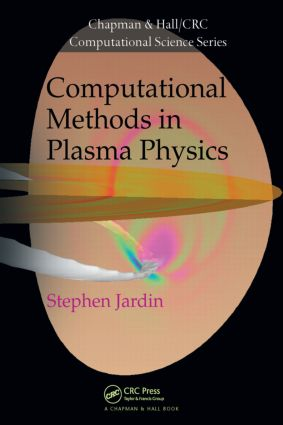# Computational Methods in Plasma Physics

## 1st Edition

CRC Press

372 pages | 61 B/W Illus.

Hardback: 9781439810217
pub: 2010-06-02
\$115.00
x
eBook (VitalSource) : 9780429075537
pub: 2010-06-02
from \$28.98

FREE Standard Shipping!

### Description

Assuming no prior knowledge of plasma physics or numerical methods, Computational Methods in Plasma Physics covers the computational mathematics and techniques needed to simulate magnetically confined plasmas in modern magnetic fusion experiments and future magnetic fusion reactors. Largely self-contained, the text presents the basic concepts necessary for the numerical solution of partial differential equations.

Along with discussing numerical stability and accuracy, the author explores many of the algorithms used today in enough depth so that readers can analyze their stability, efficiency, and scaling properties. He focuses on mathematical models where the plasma is treated as a conducting fluid, since this is the most mature plasma model and most applicable to experiments. The book also emphasizes toroidal confinement geometries, particularly the tokamak—a very successful configuration for confining a high-temperature plasma. Many of the basic numerical techniques presented are also appropriate for equations encountered in a higher-dimensional phase space.

One of the most challenging research areas in modern science is to develop suitable algorithms that lead to stable and accurate solutions that can span relevant time and space scales. This book provides an excellent working knowledge of the algorithms used by the plasma physics community, helping readers on their way to more advanced study.

### Reviews

This book provides a comprehensive and self-contained introduction to the computational methods used in plasma physics. The author successfully familiarizes readers with the basic concepts of numerical methods for partial differential equations and conjoins these methods with the magnetohydrodynamic equations that are used in plasma physics. … The extensive treatment of the material, the problems in each chapter, and the accurate topic presentation in this book make it an appropriate textbook for graduate students in physics and engineering with no prior knowledge of plasma physics or numerical mathematics. … great textbook on a highly complex scientific subject. I highly recommend this book …

Computing Reviews, January 2011

Introduction to Magnetohydrodynamic Equations

Introduction

Magnetohydrodynamic (MHD) Equations

Characteristics

Introduction to Finite Difference Equations

Introduction

Implicit and Explicit Methods

Errors

Consistency, Convergence, and Stability

Von Neumann Stability Analysis

Accuracy and Conservative Differencing

Finite Difference Methods for Elliptic Equations

Introduction

One Dimensional Poisson’s Equation

Two Dimensional Poisson’s Equation

Matrix Iterative Approach

Physical Approach to Deriving Iterative Methods

Multigrid Methods

Krylov Space Methods

Finite Fourier Transform

Plasma Equilibrium

Introduction

The Meaning of Ψ

Exact Solutions

Variational Forms of the Equilibrium Equation

Experimental Equilibrium Reconstruction

Magnetic Flux Coordinates in a Torus

Introduction

Preliminaries

Magnetic Field, Current, and Surface Functions

Constructing Flux Coordinates from Ψ(R, Z)

Inverse Equilibrium Equation

Diffusion and Transport in Axisymmetric Geometry

Introduction

Basic Equations and Orderings

Equilibrium Constraint

Time Scales

Numerical Methods for Parabolic Equations

Introduction

One Dimensional Diffusion Equations

Multiple Dimensions

Methods of Ideal MHD Stability Analysis

Introduction

Basic Equations

Variational Forms

Cylindrical Geometry

Toroidal Geometry

Numerical Methods for Hyperbolic Equations

Introduction

Explicit Centered-Space Methods

Explicit Upwind Differencing

Limiter Methods

Implicit Methods

Spectral Methods for Initial Value Problems

Introduction

Orthogonal Expansion Functions

Non-Linear Problems

Time Discretization

Implicit Example: Gyrofluid Magnetic Reconnection

The Finite Element Method

Introduction

Ritz Method in One Dimension

Galerkin Method in One Dimension

Finite Elements in Two Dimensions

Eigenvalue Problems

Bibliography

Index

A Summary appears at the end of each chapter.

Stephen Jardin is a Principal Research Physicist at the Princeton Plasma Physics Laboratory, where he is head of the Theoretical Magnetohydrodynamics Division and co-head of the Computational Plasma Physics Group. He is also a professor in the Department of Astrophysical Sciences at Princeton University and Director and Principal Investigator of the SciDAC Center for Extended Magnetohydrodynamic Modeling. Dr. Jardin is the primary developer of several widely used fusion plasma simulation codes and is currently a U.S. member of the International Tokamak Physics Activity that advises the physics staff of ITER, the world’s largest fusion experiment.